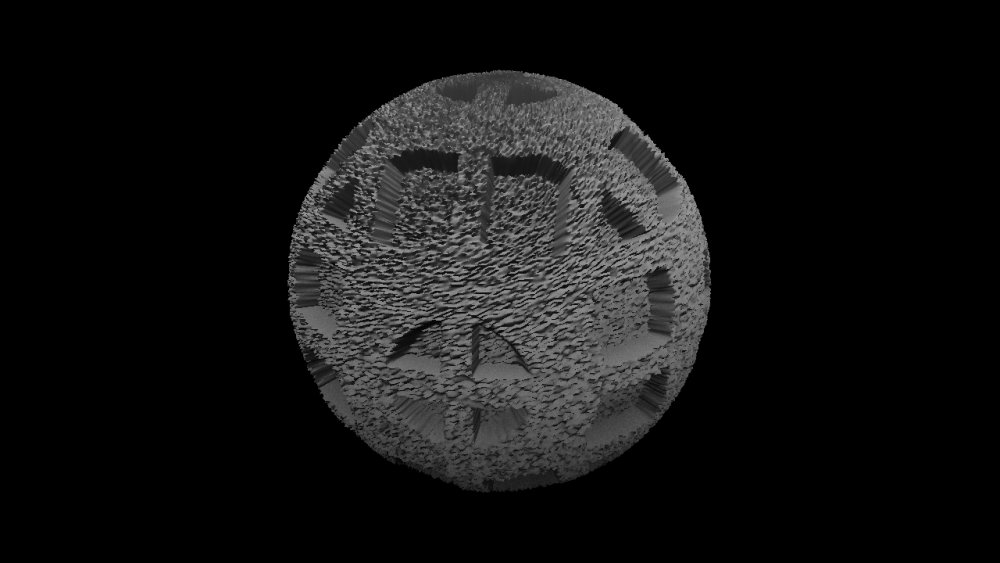## Method

This node displaces the vertices of a mesh using noise, fractal noise, a grid, an input image or a video node.

ExampleExample Bin Example Project

## Transform

Name Description
Position X Move its position along the x-axis in local space.
Position Y Move its position along the y-axis in local space.
Position Z Move its position along the z-axis in local space.
Rotation Pitch Rotate the object about the y-axis.
Rotation Bank Rotate the object about the z-axis.
Scale X Scale along the x-axis.
Scale Y Scale along the y-axis.
Scale Z Scale along the z-axis.

## Inherit Transform Channels

Toggle which transform channels should be inherited. By default, all are on.

## Attributes

Name Description
Blend Amount How much the deformer affects the mesh.
Subdivision Order Toggle whether the deformer is added before or after the object is subdivided.
Subdivision Level The subdivision level at which the deformer is applied.
Weightmap Use a weight map to determine where the deformer is more or less effective.
Vertex Source Mode How vertexes are selected to be used by the deformer.
• Vertex Positions, Vertexes are chosen based on their positions in the scene.
• Chunks, Vertexes are chosen based on the enclosed section of the mesh they are used.
Deformer Transform Space Select which transform space should be used to calculate the deformations.
• Local, Deformations are calculated in the Local Space of the object.
• World, Deformations are calculated in the World Space of the scene.
Update Time Mode How the deformer updates with relation to the time code.
• Locked To Timecode, The deformer deformations are locked to the time code and the same time will always yield the same deformations.
• Running / Looping, The deformer deformations are disconnected from the time code and will loop seamlessly at the end of the layer.
Displacement Amount How much the deformer displaces the original mesh.
Noise Amount The amount of noise that is applied to the deformer.
Fractal Noise Amount The amount of fractal noise that is applied to the deformer.
Fractal Noise Scale The scale of the fractal noise that is applied to the deformer.
Fractal Noise Threshold The threshold for the fractal noise.
Grid Amount How much the deformer is limited to a grid.
Texture Amount How much a video input into the node or an image selected in the image attribute affects the deformer.
Max Displacement Maximum distance the deformer can displace the mesh.
Animation Rate Speed the deformer is animated at.
Num Octaves How many time the noise generation is iterated.
Displacement Direction Change which direction the deformer displaces the objects mesh.
• Normal, The mesh will be displaced in the direction of the vertex normal’s.
• Spherical, The mesh will be displaced in a spherical direction from the object’s origin.
• Planar, all vertexes are displaced in the same direction.

## Falloff

Name Description
Falloff Enabled Enable falloff from the position of the deformer.
Falloff Shape Select which shape to use to draw falloff from.
• Sphere, Falloff is drawn outward from a central point, forming a spherical falloff.
• Cylinder – X/Y/Z, Falloff is drawn outward from a line, forming a cylindrical falloff.
• Plane – X/Y/Z, Falloff is drawn outward from a plane.
Falloff Range Limits the range the deformer is effective at.
Falloff Inner Range The inner range from which the falloff is calculated from.
Falloff Curve Power How quickly the falloff drops to 0 at the outer falloff.
Invert Falloff Invert the direction of the falloff.
Falloff Curve Visualises the falloff curve, from being completely in effective to not at all.

## Inputs

Name Description Typical Node Input
Image Node Image used to displace the image. Video Loader
Grid Node A node used to control the transformation values of the grid attribute. Null Node
Video Processing Node Use a video input to modify the deformer. Video Loader
Falloff Node Use an input object to control the transformation values of the falloff. Null
Generated Weightmap Add a weightmap to control the strength of the deformer depending on the weightmaps vertex data. Generate Weightmap Deformer
Transform Modifier Links all transform properties to the input node. Null
Target Node Always faces the x-axis towards the inputs anchor point. Null
Local Transform Override Override the transformation values of the node, relative to its parent. Null

## Outputs

There are no outputs from this node.